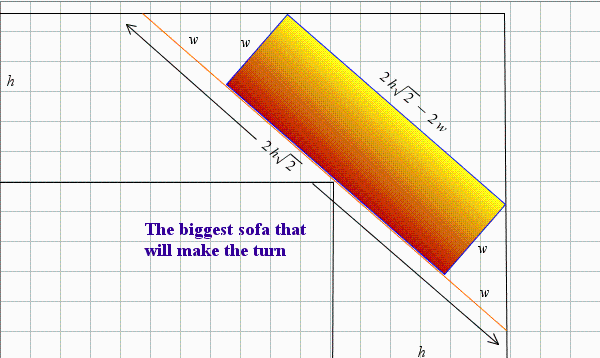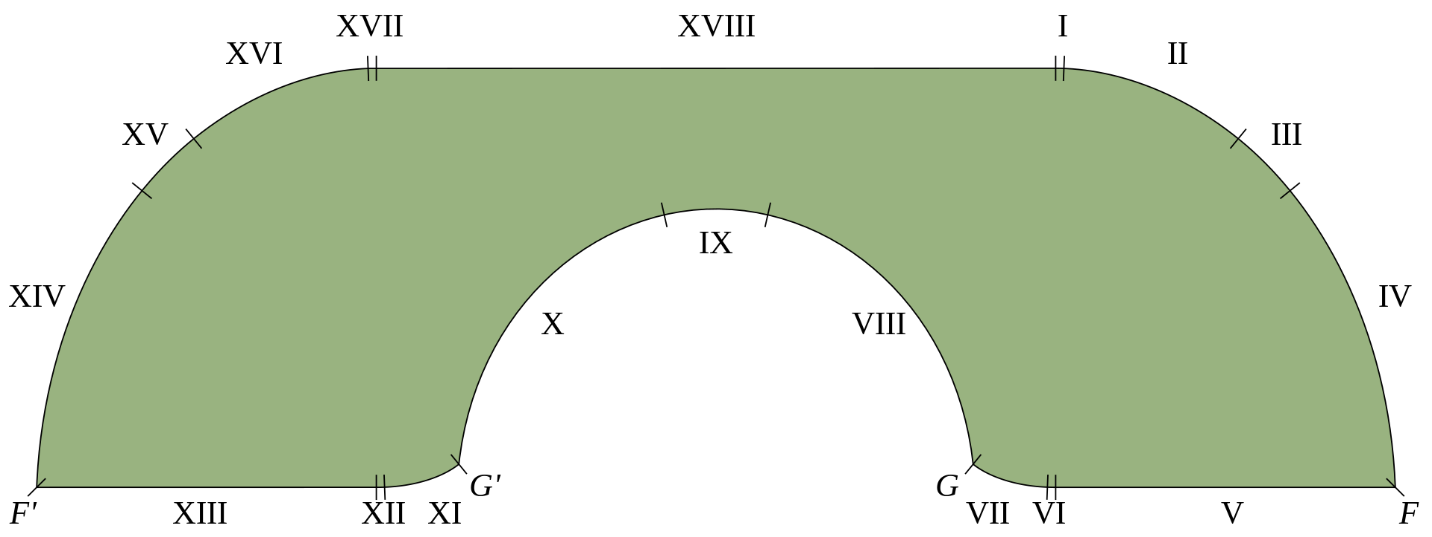## The Moving Sofa Problem

Maple Learn

Last week, one of our Maple Learn developers, Valerie McKay-Crites, published a Maple Learn document, based on the very popular Maple application by Highschool Teacher, Jason Schattman called "Just Move It Over There, Dear!".

In the Maple application, Schattman explains the math behind moving a rectangular sofa down a hallway with a 90-degree turn. In the 3D Moving Sofa Problem Estimate, Valerie uses Schattman’s math to determine the largest rectangular sofa that can be taken down a flight of stairs and down a hallway with a 90-degree turn. Both applications reminded me of how interesting the Moving Sofa Problem is, which inspired me to write a blog post about it!

If you’ve ever been tasked with moving a rectangular sofa around a 90-degree turn, you might wonder:

What is the largest sofa that can make the move?Following these steps as outlined in Schattman’s "Just Move It Over There, Dear!", will guarantee that the sofa will make the turn:

1. Measure the width of the hallway (h)
2. Measure the length (L) and width (w) of the sofa.
3. If L + 2w is comfortably less than triple the width of the hall, you'll make it!

When we work out the math exactly, we see that if the sofa's length plus double its width is less than, the sofa will make the turn!This problem is easy if we only consider rectangular sofas, however, the problem becomes significantly more complex if we consider sofas of different shapes and areas. In mathematics, this problem is known as the Moving Sofa Problem, and it is unsolved. If we look at a hallway with a 90-degree turn and legs of width 1 m (i.e. h = 1 above), the largest known sofa that can make the turn is Gerver’s Sofa which has an area of 2.2195 m2, this area is known as the Sofa Constant. Gerver’s Sofa, created in 1992, was constructed with 18 curve sections:Check out this GIF of the sofa moving through the turn. It provides some insight into why Gerver’s sofa is such an interesting shape:What is fascinating is that no mathematician has yet to prove that Gerver’s sofa is the sofa with the largest area capable of making the 90-degree turn.

The Moving Sofa Problem, is a great example of how math is embedded in our everyday lives. So, don’t stop being curious about the math around you as it can be fascinating and sometimes unproven!

If you are curious to learn more about the moving sofa problem check out this video by Numberphile, featuring Dan Romik from UC Davis: https://www.youtube.com/watch?v=rXfKWIZQIo4&t=1s﻿